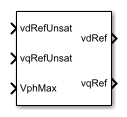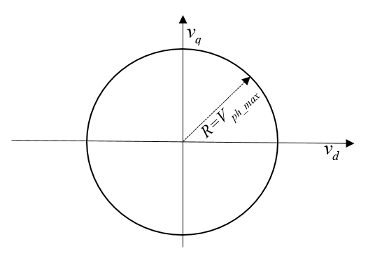# d-q Voltage Limiter

Limit voltage in the rotor direct-quadrature reference frame

• Library:
• Simscape / Electrical / Control / Protection

•## Description

The d-q Voltage Limiter block implements a voltage limiter in the rotor direct-quadrature (d-q) reference frame.

### Equations

The figure shows the circle that limits the d-q voltage vector.That is,

`$\sqrt{{v}_{d}^{2}+{v}_{q}^{2}}\le {V}_{ph_max}$`

where:

• vd is the d-axis voltage.

• vq is the q-axis voltage.

• Vph_max is the maximum phase voltage.

Three cases of voltage limiting are possible:

• d-axis prioritization

• q-axis prioritization

• d-q equivalence

If one axis is prioritized over the other axis, the constrained or saturated voltages are defined as

`${v}_{1}^{sat}=\mathrm{min}\left(\mathrm{max}\left({v}_{1}^{unsat},-{V}_{ph_max}\right),{V}_{ph_max}\right)$`

and

`${v}_{2}^{sat}=\mathrm{min}\left(\mathrm{max}\left({v}_{2}^{unsat},-{V}_{2_\mathrm{max}}\right),{V}_{2_\mathrm{max}}\right),$`

where:

• ${v}_{2_max}=\sqrt{{\left({V}_{ph_max}\right)}^{2}-{\left({v}_{1}^{sat}\right)}^{2}}$

• v1 is voltage of the prioritized axis.

• v2 is voltage of the nonprioritized axis.

If neither axis is prioritized, the constrained voltages are defined as

`${v}_{d}^{sat}=\mathrm{min}\left(\mathrm{max}\left({v}_{d}^{unsat},-{V}_{d_\mathrm{max}}\right),{V}_{d_\mathrm{max}}\right)$`

and

`${v}_{q}^{sat}=\mathrm{min}\left(\mathrm{max}\left({v}_{q}^{unsat},-{V}_{q_\mathrm{max}}\right),{V}_{q_\mathrm{max}}\right),$`

where:

• ${V}_{d_max}=\frac{{V}_{ph_max}|{v}_{d}^{unsat}|}{\sqrt{{\left({v}_{d}^{unsat}\right)}^{2}+{\left({v}_{q}^{unsat}\right)}^{2}}}$

• ${V}_{q_max}=\frac{{V}_{ph_max}|{v}_{q}^{unsat}|}{\sqrt{{\left({v}_{d}^{unsat}\right)}^{2}+{\left({v}_{q}^{unsat}\right)}^{2}}}$

## Ports

### Input

expand all

Unsaturated direct-axis reference voltage.

Example: Example

Data Types: `single` | `double`

Unsaturated quadrature-axis reference voltage.

Example: Example

Data Types: `single` | `double`

Maximum phase voltage.

Data Types: `single` | `double`

### Output

expand all

Saturated direct-axis reference voltage.

Data Types: `single` | `double`

Saturated quadrature-axis reference voltage.

Example: Example

Data Types: `single` | `double`

## Parameters

expand all

Prioritize the direct-axis, the quadrature-axis, or neither axis.

Time interval between samples. If the block is inside a triggered subsystem, inherit the sample time by setting this parameter to `-1`. If this block is in a continuous variable-step model, specify the sample time explicitly. For more information, see What Is Sample Time? and Specify Sample Time.

## Version History

Introduced in R2017b